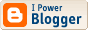## Duck to century ratios

SMarsh Oz's 1319th Test duck. 747 Oz centuries. Oz 1.77 ducks per century Ind 2.01 ducks per century. Bangladesh 9 ducks/100!

As a bit of pointless fun, let's pretend that all representatives of each country are equally good batsmen (a terrible assumption, obviously, but perhaps the tail-enders' ducks and top-order centuries will roughly cancel out in the right ratios) and apply the hazard function mentioned at the end of this post.

This hazard function is the simplest extension of the constant-hazard model, the latter saying, effectively, that a batsman always has his eye in. In the "new" model, the batsman is three times more likely to get out on nought than to get out when on some other score, but gets his eye in as soon as he's off the mark. When studying the proportion of ducks, this is an important change to make, because the constant-hazard model will under-estimate the number of ducks.

The algebra is boring and I will skip it. The probability of a duck is 3/(avg + 3), and the probability of a score less than 100 is 3/(avg + 3) + {1 - [(avg+2)/(avg+3)]98} * avg/(avg + 3). Subtract the latter expression from 1 to get the probability of scoring a century.

Throw it into an Excel formula, and you find that a duck:century ratio of 9 corresponds to an average of about 21.1, a ratio of 2.01 corresponds to an average of about 30.1, and a ratio of 1.77 corresponds to an average of about 31.1.

The actual averages are 20.6, 31.5, and 32.3 respectively.

(Edit: Fixed the exponent in the probability for a score less than 100.)

(Edit 2, 8/1/2011: The earlier edit fixed the typo in my transcription from my handwritten work to the blog, but the handwritten work was wrong. The exponent should be 99, not 98. The corresponding averages to the three duck:century ratios should be about 21.3, 30.4, and 31.4 respectively.)Subscribe to Posts [Atom]